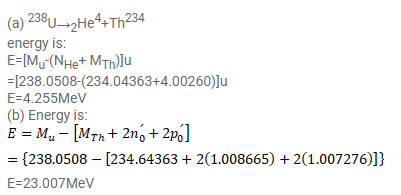# (a) Calculate the energy released if 238U emits an ????-particle.

Question:

(a) Calculate the energy released if ${ }^{238} \mathrm{U}$ emits an $\alpha$-particle. (b) Calculate the energy to be supplied to ${ }^{238} \mathrm{U}$ if two protons and two neutrons are to be emitted one by one. The atomic masses of ${ }^{238} \mathrm{U},{ }^{234} \mathrm{Th}$ and ${ }^{4} \mathrm{He}$ are $238.0508 \mathrm{u}, 234.04363 \mathrm{u}$ and 4.00260u respectively.

Solution: